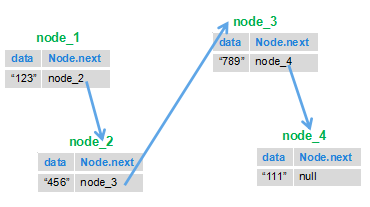# 剑指offer部分编程题

• 计算机基础
• 算法
2022年3月3日约 4857 字大约 16 分钟

## # 斐波那契数列

``````int Fibonacci(int number) {
if (number <= 0) {
return 0;
}
if (number == 1 || number == 2) {
return 1;
}
int first = 1, second = 1, third = 0;
for (int i = 3; i <= number; i++) {
third = first + second;
first = second;
second = third;
}
return third;
}
``````

``````public int Fibonacci(int n) {
if (n <= 0) {
return 0;
}
if (n == 1||n==2) {
return 1;
}

return Fibonacci(n - 2) + Fibonacci(n - 1);
}
``````

## # 跳台阶问题

a.如果两种跳法，1阶或者2阶，那么假定第一次跳的是一阶，那么剩下的是n-1个台阶，跳法是f(n-1); b.假定第一次跳的是2阶，那么剩下的是n-2个台阶，跳法是f(n-2) c.由a，b假设可以得出总跳法为: f(n) = f(n-1) + f(n-2) d.然后通过实际的情况可以得出：只有一阶的时候 f(1) = 1 ,只有两阶的时候可以有 f(2) = 2

f(1) = 1, f(2) = 2, f(3) = 3, f(4) = 5， 可以总结出f(n) = f(n-1) + f(n-2)的规律。但是为什么会出现这样的规律呢？假设现在6个台阶，我们可以从第5跳一步到6，这样的话有多少种方案跳到5就有多少种方案跳到6，另外我们也可以从4跳两步跳到6，跳到4有多少种方案的话，就有多少种方案跳到6，其他的不能从3跳到6什么的啦，所以最后就是f(6) = f(5) + f(4)；这样子也很好理解变态跳台阶的问题了。

``````int jumpFloor(int number) {
if (number <= 0) {
return 0;
}
if (number == 1) {
return 1;
}
if (number == 2) {
return 2;
}
int first = 1, second = 2, third = 0;
for (int i = 3; i <= number; i++) {
third = first + second;
first = second;
second = third;
}
return third;
}
``````

## # 变态跳台阶问题

``````int JumpFloorII(int number) {
return 1 << --number;//2^(number-1)用位移操作进行，更快
}
``````

java中有三种移位运算符：

1. “<<” : 左移运算符，等同于乘2的n次方
2. “>>”: 右移运算符，等同于除2的n次方
3. “>>>” : 无符号右移运算符，不管移动前最高位是0还是1，右移后左侧产生的空位部分都以0来填充。与>>类似。
``````int a = 16;
int b = a << 2;//左移2，等同于16 * 2的2次方，也就是16 * 4
int c = a >> 2;//右移2，等同于16 / 2的2次方，也就是16 / 4
``````

## # 二维数组查找

``````public boolean Find(int target, int [][] array) {
//基本思路从左下角开始找，这样速度最快
int row = array.length-1;//行
int column = 0;//列
//当行数大于0，当前列数小于总列数时循环条件成立
while((row >= 0)&& (column< array.length)){
if(array[row][column] > target){
row--;
}else if(array[row][column] < target){
column++;
}else{
return true;
}
}
return false;
}
``````

## # 替换空格

``````public String replaceSpace(StringBuffer str) {
StringBuffer out = new StringBuffer();
for (int i = 0; i < str.toString().length(); i++) {
char b = str.charAt(i);
if(String.valueOf(b).equals(" ")){
out.append("%20");
}else{
out.append(b);
}
}
return out.toString();
}
``````

``````public String replaceSpace(StringBuffer str) {
//return str.toString().replaceAll(" ", "%20");
//public String replaceAll(String regex,String replacement)
//用给定的替换替换与给定的regular expression匹配的此字符串的每个子字符串。
//\ 转义字符. 如果你要使用 "\" 本身, 则应该使用 "\\". String类型中的空格用“\s”表示，所以我这里猜测"\\s"就是代表空格的意思
return str.toString().replaceAll("\\s", "%20");
}
``````

## # 数值的整数次方

``````public class Solution {
boolean invalidInput=false;
public double Power(double base, int exponent) {
//如果底数等于0并且指数小于0
//由于base为double型，不能直接用==判断
if(equal(base,0.0)&&exponent<0){
invalidInput=true;
return 0.0;
}
int absexponent=exponent;
//如果指数小于0，将指数转正
if(exponent<0)
absexponent=-exponent;
//getPower方法求出base的exponent次方。
double res=getPower(base,absexponent);
//如果指数小于0，所得结果为上面求的结果的倒数
if(exponent<0)
res=1.0/res;
return res;
}
//比较两个double型变量是否相等的方法
boolean equal(double num1,double num2){
if(num1-num2>-0.000001&&num1-num2<0.000001)
return true;
else
return false;
}
//求出b的e次方的方法
double getPower(double b,int e){
//如果指数为0，返回1
if(e==0)
return 1.0;
//如果指数为1，返回b
if(e==1)
return b;
//e>>1相等于e/2，这里就是求a^n =（a^n/2）*（a^n/2）
double result=getPower(b,e>>1);
result*=result;
//如果指数n为奇数，则要再乘一次底数base
if((e&1)==1)
result*=b;
return result;
}
}
``````

``````// 使用累乘
public double powerAnother(double base, int exponent) {
double result = 1.0;
for (int i = 0; i < Math.abs(exponent); i++) {
result *= base;
}
if (exponent >= 0)
return result;
else
return 1 / result;
}
``````

## # 调整数组顺序使奇数位于偶数前面

``````public class Solution {
public void reOrderArray(int [] array) {
//如果数组长度等于0或者等于1，什么都不做直接返回
if(array.length==0||array.length==1)
return;
//oddCount：保存奇数个数
//oddBegin：奇数从数组头部开始添加
int oddCount=0,oddBegin=0;
//新建一个数组
int[] newArray=new int[array.length];
//计算出（数组中的奇数个数）开始添加元素
for(int i=0;i<array.length;i++){
if((array[i]&1)==1) oddCount++;
}
for(int i=0;i<array.length;i++){
//如果数为基数新数组从头开始添加元素
//如果为偶数就从oddCount（数组中的奇数个数）开始添加元素
if((array[i]&1)==1)
newArray[oddBegin++]=array[i];
else newArray[oddCount++]=array[i];
}
for(int i=0;i<array.length;i++){
array[i]=newArray[i];
}
}
}
``````

## # 链表中倒数第k个节点

2. p1的下一步是走第k步，这个时候，p2开始一起动了。至于为什么p2这个时候动呢？看下面的分析。
3. 当p1走到链表的尾部时，即p1走了n步。由于我们知道p2是在p1走了k-1步才开始动的，也就是说p1和p2永远差k-1步。所以当p1走了n步时，p2走的应该是在n-(k-1)步。即p2走了n-k+1步，此时巧妙的是p2正好指向的是规律一的倒数第k个结点处。 这样是不是很好理解了呢？

``````/*
//链表类
public class ListNode {
int val;
ListNode next = null;

ListNode(int val) {
this.val = val;
}
}*/

//时间复杂度O(n),一次遍历即可
public class Solution {
public ListNode FindKthToTail(ListNode head,int k) {
ListNode pre=null,p=null;
//两个指针都指向头结点
//记录k值
int a=k;
//记录节点的个数
int count=0;
//p指针先跑，并且记录节点数，当p指针跑了k-1个节点后，pre指针开始跑，
//当p指针跑到最后时，pre所指指针就是倒数第k个节点
while(p!=null){
p=p.next;
count++;
if(k<1){
pre=pre.next;
}
k--;
}
//如果节点个数小于所求的倒数第k个节点，则返回空
if(count<a) return null;
return pre;

}
}
``````

## # 反转链表``````/*
public class ListNode {
int val;
ListNode next = null;

ListNode(int val) {
this.val = val;
}
}*/
public class Solution {
ListNode next = null;
ListNode pre = null;
//保存要反转到头来的那个节点
//要反转的那个节点指向已经反转的上一个节点
//上一个已经反转到头部的节点
//一直向链表尾走
}
return pre;
}
}
``````

## # 合并两个排序的链表

1. 假设我们有两个链表 A,B；
2. A的头节点A1的值与B的头结点B1的值比较，假设A1小，则A1为头节点；
3. A2再和B1比较，假设B1小,则，A1指向B1；
4. A2再和B2比较。。。。。。。 就这样循环往复就行了，应该还算好理解。

``````/*
public class ListNode {
int val;
ListNode next = null;

ListNode(int val) {
this.val = val;
}
}*/
public class Solution {
public ListNode Merge(ListNode list1,ListNode list2) {
//list1为空，直接返回list2
if(list1 == null){
return list2;
}
//list2为空，直接返回list1
if(list2 == null){
return list1;
}
ListNode current = null;
//当list1和list2不为空时
while(list1!=null && list2!=null){
//取较小值作头结点
if(list1.val <= list2.val){
}else{
current.next = list1;
//current节点保存list1节点的值因为下一次还要用
current = list1;
}
//list1指向下一个节点
list1 = list1.next;
}else{
}else{
current.next = list2;
//current节点保存list2节点的值因为下一次还要用
current = list2;
}
//list2指向下一个节点
list2 = list2.next;
}
}
if(list1 == null){
current.next = list2;
}else{
current.next = list1;
}
}
}
``````

``````public ListNode Merge(ListNode list1,ListNode list2) {
if(list1 == null){
return list2;
}
if(list2 == null){
return list1;
}
if(list1.val <= list2.val){
list1.next = Merge(list1.next, list2);
return list1;
}else{
list2.next = Merge(list1, list2.next);
return list2;
}
}
``````

## # 用两个栈实现队列

``````//左程云的《程序员代码面试指南》的答案
import java.util.Stack;

public class Solution {
Stack<Integer> stack1 = new Stack<Integer>();
Stack<Integer> stack2 = new Stack<Integer>();

//当执行push操作时，将元素添加到stack1
public void push(int node) {
stack1.push(node);
}

public int pop() {
//如果两个队列都为空则抛出异常,说明用户没有push进任何元素
if(stack1.empty()&&stack2.empty()){
throw new RuntimeException("Queue is empty!");
}
//如果stack2不为空直接对stack2执行pop操作，
if(stack2.empty()){
while(!stack1.empty()){
//将stack1的元素按后进先出push进stack2里面
stack2.push(stack1.pop());
}
}
return stack2.pop();
}
}
``````

## # 栈的压入,弹出序列

【思路】借用一个辅助的栈，遍历压栈顺序，先讲第一个放入栈中，这里是1，然后判断栈顶元素是不是出栈顺序的第一个元素，这里是4，很显然1≠4，所以我们继续压栈，直到相等以后开始出栈，出栈一个元素，则将出栈顺序向后移动一位，直到不相等，这样循环等压栈顺序遍历完成，如果辅助栈还不为空，说明弹出序列不是该栈的弹出顺序。

…. 依次执行，最后辅助栈为空。如果不为空说明弹出序列不是该栈的弹出顺序。

``````import java.util.ArrayList;
import java.util.Stack;
//这道题没想出来，参考了Alias同学的答案：https://www.nowcoder.com/questionTerminal/d77d11405cc7470d82554cb392585106
public class Solution {
public boolean IsPopOrder(int [] pushA,int [] popA) {
if(pushA.length == 0 || popA.length == 0)
return false;
Stack<Integer> s = new Stack<Integer>();
//用于标识弹出序列的位置
int popIndex = 0;
for(int i = 0; i< pushA.length;i++){
s.push(pushA[i]);
//如果栈不为空，且栈顶元素等于弹出序列
while(!s.empty() &&s.peek() == popA[popIndex]){
//出栈
s.pop();
//弹出序列向后一位
popIndex++;
}
}
return s.empty();
}
}
``````